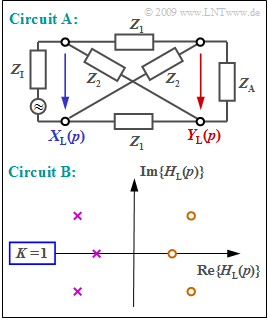# Difference between revisions of "Aufgaben:Exercise 3.4Z: Various All-Pass Filters"All-pass filter in two different variants
$Z_{\rm I}$:  Internal resistance of the source
$Z_{\rm A}$:  Terminating resistor

We first assume a two-port network with the following transfer function:

$$H_{\rm L}(p)= \frac {1-{p}/{A}} {1+{p}/{A}}.$$

From this is to be determined the conventional Fourier frequency response

$$H(f) = {\rm e}^{-a(f)\hspace{0.05cm}}\cdot {\rm e}^{- \hspace{0.05cm}{\rm j} \hspace{0.05cm}\cdot \hspace{0.05cm}b(f)},$$

which is representable

• by the attenuation function  $a(f)$
• and the phase function  $b(f)$.

The upper diagram shows a so-called  "all-pass circuit"  where the complex resistance  $Z_1$  denotes an inductance and the complex resistance  $Z_2$  denotes a capacitance:

$$Z_1 = p \cdot L\hspace{0.05cm},\hspace{0.2cm}Z_2 = \frac{1}{p \cdot C}\hspace{0.05cm}.$$

For reflection-free adaptation at the input and output with

$$Z_{\rm I}=Z_{\rm A} = \sqrt{Z_1 \cdot Z_2} = \sqrt{{L}/{C}},$$

the following holds for the  $p$–transfer function of circuit  $\rm A$  (see upper diagram):

$$H_{\rm L}(p)= \frac {Y_{\rm L}(p)} {X_{\rm L}(p)}= \frac {Z_2-Z_1} {Z_1+2 \cdot \sqrt{Z_1 \cdot Z_2}+Z_2}\hspace{0.05cm}.$$

Circuit  $\rm B$  is defined by the pole–zero diagram of the  $p$–transfer function.  It is characterized by the fact that

• all poles  (in the left $p$–half-plane)
• are located in a mirror-imaged manner with respect to the zeros  (in the right half-plane).

### Questions

1

Specify the zero  $p_{\rm o}$  and the pole  $p_{\rm x}$  of  $H_{\rm L}(p)= (1 -p/A)/(1 +p/A)$ .  What is the constant factor  $K$?

 $K \ = \$ $p_{\rm o} \ = \$ $\ \cdot A$ $p_{\rm x} \ = \$ $\ \cdot A$

2

Compute the attenuation function  $a(f)$.  Which statements are true?

 The attenuation function  $a(f)$  exhibits low-pass filter behavior. The attenuation function  $a(f)$  is constant. The above result is generally valid for  $p_{\rm x} = - p_{\rm o}$.

3

Compute the phase response  $b(f)$.  What are the phase values for the given frequencies?

 $b(f = A/2 \pi) \ = \$ $\ \rm deg$ $b(f = A/ \pi)\ = \$ $\rm deg$ $b(f → ∞) \ = \$ $\rm deg$

4

Compute the  $p$–transfer function of circuit  $\rm A$.  What statements can be derived from this?

 The attenuation  $a(f)$  is constantly equal to  $0$  (Np). The phase  $b(f)$  increases linearly with frequency  $f$ . $b(f)$  is the Hilbert transform of  $a(f)$.

5

What statements can be derived from the pole–zero diagram of circuit  $\rm B$ ?

 The attenuation  $a(f)$  is constant. The following holds for the phase function:  $b(f =0) =0$.

### Solution

#### Solution

(1)  Transforming the given  $p$–transfer function yields

$$H_{\rm L}(p)= \frac {1-{p}/{A}} {1+{p}/{A}}= -1 \cdot \frac {p-A} {p+A}\hspace{0.3cm}\Rightarrow \hspace{0.3cm}\hspace{0.15cm} \underline{K =- 1}, \hspace{0,2cm} \hspace{0.15cm} \underline{p_{\rm o}/A = 1} ,\hspace{0,2cm} \hspace{0.15cm} \underline{ p_{\rm x}/A = -1} \hspace{0.05cm} .$$

(2)  Statements 2 and 3  are correct:

• If  $p = {\rm j} \cdot 2 \pi f$ is set, the following is obtained:
$$H(f)= \frac {1-{\rm j \cdot 2\pi \it f}/A} {1+{\rm j \cdot 2\pi \it f}/A}\hspace{0.05cm} .$$
• The magnitude of a quotient is equal to the quotient of the magnitudes:
$$|H(f)|= \frac {|1-{{\rm j} \cdot 2\pi f}/A|} {|1+{\rm j \cdot 2\pi \it f}/A|}= \frac {\sqrt{1+(2\pi f/A)^2}} {\sqrt{1+(2\pi f/A)^2}}= 1\hspace{0.3cm} \Rightarrow \hspace{0.3cm} a(f)= -{\rm ln} \hspace{0.1cm} |H(f)|= 0\hspace{0.2cm}({\rm Np \hspace{0.2cm}or \hspace{0.2cm}dB})\hspace{0.05cm} .$$
• However,  $\text{statement 3}$  is also correct as can be seen from the theory page  "Graphical determination of attenuation".

(3)  The phase function  $b(f)$  can be computed as follows:

$$b(f)= -{\rm arc} \hspace{0.1cm} H(f) \hspace{0.25cm} = \hspace{0.2cm} {\rm arctan } \hspace{0.1cm} ({2\pi f}/{A}) - {\rm arctan } \hspace{0.1cm} ({-2\pi f}/{A}) = 2 \cdot {\rm arctan } \hspace{0.1cm} ({2\pi f}/{A}),$$
$$b(f= {A}/{2\pi})= 2 \cdot {\rm arctan } \hspace{0.1cm}(1) = 2 \cdot 45^\circ \hspace{0.15cm} \underline{ = 90^\circ}\hspace{0.05cm},$$
$$b(f= {A}/{\pi})=2 \cdot {\rm arctan } \hspace{0.1cm}(2) = 2 \cdot 63.4^\circ \hspace{0.15cm} \underline{= 126.8^\circ}\hspace{0.05cm} ,$$
$$b(f \rightarrow \infty)=2 \cdot {\rm arctan } \hspace{0.1cm}(\infty) = 2 \cdot 90^\circ \hspace{0.15cm} \underline{= 180^\circ}\hspace{0.05cm} .$$
• The same results are obtained following the approach according to the page  "Graphical determination of phase"  in the theory part.

(4)  Only statement 1  is correct:

• The given  $p$–transfer function can be expressed as follows:
$$H_{\rm L}(p)= \frac {Z_2-Z_1} {Z_1+2 \cdot \sqrt{Z_1 \cdot Z_2}+Z_2}= \frac {(\sqrt{Z_2}-\sqrt{Z_1})(\sqrt{Z_2}+\sqrt{Z_1})} {(\sqrt{Z_2}+\sqrt{Z_1})^2}= \frac {\sqrt{Z_2}-\sqrt{Z_1}} {\sqrt{Z_2}+\sqrt{Z_1}}\hspace{0.05cm}.$$
• Furthermore,  considering  $Z_1 = p \cdot L$  and  $Z_2 = 1/(p \cdot C)$  the following is obtained:
$$H_{\rm L}(p)= \frac {\sqrt{{1}/(pC)}-\sqrt{pL}} {\sqrt{{1}/(pC)}+\sqrt{pL}} = \frac {1- p \cdot \sqrt{LC}} {1+ p \cdot \sqrt{LC}} = -1 \cdot \frac {p-\sqrt{{1}/(LC)}} {p+\sqrt{{1}/(LC)}}\hspace{0.3cm} \Rightarrow \hspace{0.3cm}A = \sqrt{{1}/(LC)}: \hspace{0.2cm}H_{\rm L}(p)= -1 \cdot \frac {p-A} {p+A}\hspace{0.05cm}.$$
• The same transfer function as computed in subtask  (1)  is obtained.

From this it follows that only statement 1 is correct:

• The attenuation curve is  $a(f) = 0\ \rm (Np)$.  No frequency is attenuated or amplified.  Therefore, it is called an "all-pass filter".
• The second statement is false.  The phase response  $b(f)$  is not linear but rather curved as computed in subtask  (3) .
• The Hilbert transform of the constant  $a(f) = 0$  should result in the phase function  $b(f) = 0$  as shown in the theory part.  That is:  statement 3 is false.
• The attenuation function  $a(f)$  and phase function  $b(f)$  are related to each other via the Hilbert transformation only for minimum-phase systems.
• However, in such a minimum–phase system, all poles and zeros lie in the left $p$–half-plane which is not true here
⇒   an all-pass filter is not a minimum–phase system.

(5)  Both statements  are correct:

• As already determined in subtask  (2):   A constant attenuation arises as a result whenever there is a corresponding zero in the right half-plane for each pole in the left $p$–half-plane   ⇒   circuit  $\rm B$  also exhibits all-pass filter charakteristics.
• Since  $b(f)$  is always an asymmetric function,  $b(f= 0) = 0$  holds in general.  That is, for any spectral function  $H(f)$ whose inverse Fourier transform  ("impulse response")  is real.